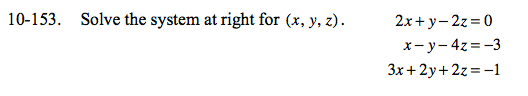Home > CCA2 > Chapter 10 > Lesson 10.3.1 > Problem10-153

10-153.
1. Solve the system at right for (x, y, z). Homework Help ✎

2. 2x + y − 2z = 0
xy − 4z = −3
3x + 2y + 2z = −1Add the first and second equation to eliminate the y-term.

Multiply the second equation by 2 and then add it to the last equation.

Use your equations from Steps 1 and 2 to solve a system with x and z.
Then use your x- and z-values to solve for y in any of the three given equations.